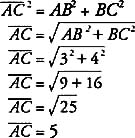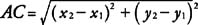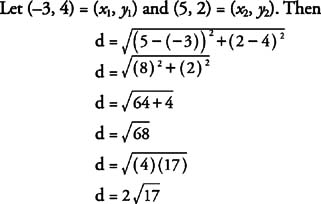## Distance Formula

In Figure 1A is (2, 2), B is (5, 2), and C is (5, 6) .Figure 1 Finding the distance from A to C.

To find AB or BC, only simple subtracting is necessary.To find AC, though, simply subtracting is not sufficient. Triangle ABC is a right triangle with AC the hypotenuse. Therefore, by the Pythagorean Theorem,If A is represented by the ordered pair ( x 1y 1) and C is represented by the ordered pair ( x 2y 2), then AB = ( x 2 − x 1) and BC = ( y 2 − y 1).

ThenThis is stated as a theorem.

Theorem 101: If the coordinates of two points are ( x 1y 1) and ( x 2y 2), then the distance, d, between the two points is given by the following formula (Distance Formula).Example 1: Use the Distance Formula to find the distance between the points with coordinates (−3, 4) and (5, 2).Example 2: A triangle has vertices A(12,5), B(5,3), and C(12, 1). Show that the triangle is isosceles.

By the Distance Formula,Because AB = BC, triangle ABC is isosceles.